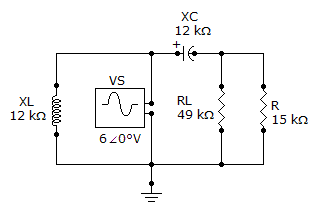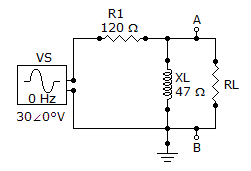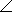# Electrical Engineering - Circuit Theorems in AC Analysis

### Exercise :: Circuit Theorems in AC Analysis - General Questions

11.

If two currents are in the same direction at any instant of time in a given branch of a circuit, the net current at that instant

 A. is zero B. is the sum of the two currents C. is the difference between the two currents D. cannot be determined

Explanation:

No answer description available for this question. Let us discuss.

12.

The Thevenin equivalent voltage is

 A. equal to the source voltage B. the same as the load voltage C. the open circuit voltage D. none of the above

Explanation:

No answer description available for this question. Let us discuss.

13.

Referring to the given circuit, LA. must be in parallel with RL B. must be placed in parallel with VS C. must have a reactance equal to XC D. has no effect on the result

Explanation:

No answer description available for this question. Let us discuss.

14.

Referring to the given circuit, find ZTH for the part of the circuit that is external to RL.A. 12921.4°B. 43.768.6°C. 43.721.4°D. 12.968.6°Explanation:

No answer description available for this question. Let us discuss.

15.

In order to get maximum power transfer from a capacitive source, the load must

 A. have a capacitive reactance equal to circuit resistance B. have an impedance that is the complex conjugate of the source impedance C. be as capacitive as it is inductive D. none of the above